# Set Theory Tutorial | Problems, Formulas, Examples

Set theory has its own notations and symbols that can seem unusual for many. In this tutorial, we look at some solved examples to understand how set theory works and the kind of problems it can be used to solve.

#### Definition

A set is a collection of objects.

It is usually represented in flower braces.

For example:
Set of natural numbers           = {1,2,3,…..}
Set of whole numbers             = {0,1,2,3,…..}

Each object is called an element of the set.

The set that contains all the elements of a given collection is called the universal set and is represented by the symbol ‘µ’, pronounced as ‘mu’.

For two sets A and B,

• n(AᴜB) is the number of elements present in either of the sets A or B.
• n(A∩B) is the number of elements present in both the sets A and B.
• n(AᴜB) = n(A) + (n(B) – n(A∩B)

For three sets A, B and C,

• n(AᴜBᴜC) = n(A) + n(B) + n(C) – n(A∩B) – n(B∩C) – n(C∩A) + n(A∩B∩C)

Consider the following example:

Question: In a class of 100 students, 35 like science and 45 like math. 10 like both. How many like either of them and how many like neither?

Solution:

Total number of students, n(µ) = 100

Number of science students, n(S) = 35

Number of math students, n(M) = 45

Number of students who like both, n(M∩S) = 10

Number of students who like either of them,

n(MᴜS) = n(M) + n(S) – n(M∩S)

→ 45+35-10 = 70

Number of students who like neither = n(µ) – n(MᴜS) = 100 – 70 = 30

The easiest way to solve problems on sets is by drawing Venn diagrams, as shown below.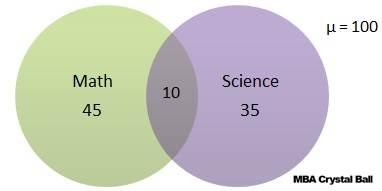As it is said, one picture is worth a thousand words. One Venn diagram can help solve the problem faster and save time. This is especially true when more than two categories are involved in the problem.

Let us see some more solved examples.

Problem 1: There are 30 students in a class. Among them, 8 students are learning both English and French. A total of 18 students are learning English. If every student is learning at least one language, how many students are learning French in total?

Solution:

The Venn diagram for this problem looks like this.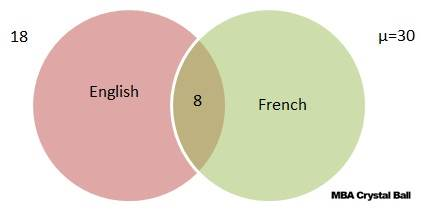Every student is learning at least one language. Hence there is no one who fall in the category ‘neither’.

So in this case, n(EᴜF) = n(µ).

It is mentioned in the problem that a total of 18 are learning English. This DOES NOT mean that 18 are learning ONLY English. Only when the word ‘only’ is mentioned in the problem should we consider it so.

Now, 18 are learning English and 8 are learning both. This means that 18 – 8 = 10 are learning ONLY English.

n(µ) = 30, n(E) = 10

n(EᴜF) = n(E) + n(F) – n(E∩F)

30 = 18+ n(F) – 8

n(F) = 20

Therefore, total number of students learning French = 20.

Note: The question was only about the total number of students learning French and not about those learning ONLY French, which would have been a different answer, 12.

Finally, the Venn diagram looks like this.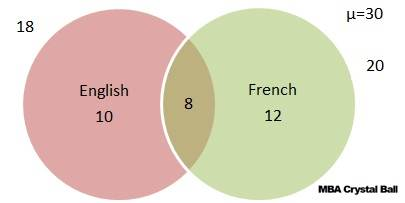Problem 2: Among a group of students, 50 played cricket, 50 played hockey and 40 played volley ball. 15 played both cricket and hockey, 20 played both hockey and volley ball, 15 played cricket and volley ball and 10 played all three. If every student played at least one game, find the number of students and how many played only cricket, only hockey and only volley ball?

Solution:

n(C) = 50, n(H) = 50, n(V) = 40

n(C∩H) = 15

n(H∩V) = 20

n(C∩V) = 15

n(C∩H∩V) = 10

No. of students who played at least one game

n(CᴜHᴜV) = n(C) + n(H) + n(V) – n(C∩H) – n(H∩V) – n(C∩V) + n(C∩H∩V)

= 50 + 50 + 40 – 15 – 20 – 15 + 10

Total number of students = 100.

Let a denote the number of people who played cricket and volleyball only.
Let b denote the number of people who played cricket and hockey only.
Let c denote the number of people who played hockey and volleyball only.
Let d denote the number of people who played all three games.

Accordingly, d = n (CnHnV) = 10

Now, n(CnV) = a + d = 15

n(CnH) = b + d = 15

n(HnV) = c + d = 20

Therefore, a = 15 – 10 = 5 [cricket and volleyball only]

b = 15 – 10 = 5 [cricket and hockey only]

c = 20 – 10 = 10 [hockey and volleyball only]

No. of students who played only cricket = n(C) – [a + b + d] = 50 – (5 + 5 + 10) = 30

No. of students who played only hockey = n(H) – [b + c + d] = 50 – ( 5 + 10 + 10) = 25

No. of students who played only volley ball = n(V) – [a + c + d] = 40 – (10 + 5 + 10) = 15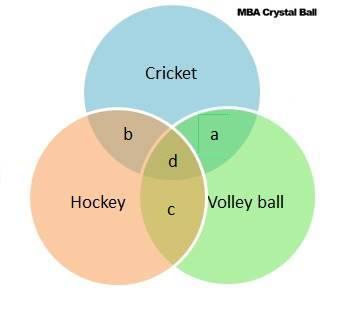Alternatively, we can solve it faster with the help of a Venn diagram.

The Venn diagram for the given information looks like this.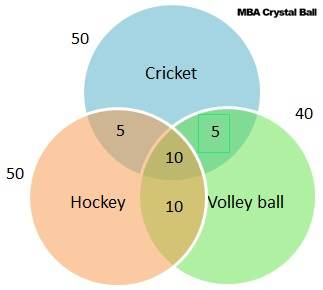Subtracting the values in the intersections from the individual values gives us the number of students who played only one game.

## Set Theory Quiz: Solve these problems for practice

MBA Song | Start here | Success stories | Reality check | Knowledgebase | Scholarships | Services### 13 thoughts on “Set Theory Tutorial | Problems, Formulas, Examples”

1. Can anyone help me and solve this question. In a bank of 320 staffs, 120 speak French, 140 speak English, 170 speak Arabic, 50 speak both French and English, 35 speak both English and Arabic, 40 speak both French and Arabic. Required ; (a) Determine the number of staffs who speak all the three languages. Thanks

• n(U) = 320, //n(F)= 120, n(E)= 140, n(A)= 170,// n(F n E)= 50, n(E n A)= 35, n(F n A)= 40// n(F n E n A)=?.// So let n(F n E n A)= X.
THE FORMULA STATED THAT
n(U) = n(F) + n(E) + n(A) – n(F n E) – n(F n A) – n(E n A) + n(F n E n A) where n(F n E n A) is X
320 = 120 + 140 + 170 – 50 -35 + 40 + X
320 = 430 – 125 + X
320 =305 + X
320 – 305 =X
15 = X
X = 15, Where X is the number of staffs who spokes all the three languages.
So the number of staffs who spokes all the three subject are 15.

2. Any body please help me to solve this problem

If A = {X:X is a prime number between 4 and 16} list the subset with exactly two elements

• here we have A be set of prime numbers between 4 and 16 so the set be X = {5,7,11,13}
SUBSETS with exactly two elements in above set X = {5,7},{5,11},{5,13},{7,11},{7,13},{11,13}

• Data given
Total no of staffs=320
Let F be number of staffs who speak French , E for English And A for Arabic
n(F)=120
n(E)=140
n(A)=170
n(FnE)=50
n(EnA)=35
n(FnA)=40
n(FnEnA)=?
From
n(FuEuA)=n(F)+n(E)+n(A)_n(FnE)_n(EnA)_n(AnF)+n(FnEnA)
320=120+140+170_50_35_40+n(FnEnA)
320=430_125
320_305=15
So
n(FnEnA)=15
So number of staffs who speak both languages are 15 staffs

• A={5,7,11,13}
Only two elements Subsets are:
{5,7},{5,11},{5,13},{7,11},{7,13} {11,13}

3. please any help? Out of 45 students questioned, 42 like mathematics or english or both, 27 students like maths and 22 like english.
1) who didn’t like any of the subjects
2)who like maths only
3)both maths and english

• HERE
we have the total number of students questioned (U)=45
the number of students like either mathematics or english n(MuE)=42
the no. of students like mathematics n(M)=27
the no.of students like english n(E)=22

1)the no.of students didn’t like any subject= U-n(MuE) =45-42=3
3)the no.of students like both maths and english = x
n(MuE)=n(M)+n(E)-x
42=27+22-x
42=49-x
x=49-42=7
2)who like math only =n(M)-x =27-7=20

4. I think you are right. I want to explain my answer in simple way.

170+140+120=430
Total=320
430-320=110
Common=35+50+40=125
125-110=15

5. possible subsets of set with 13 elements=2^13=8,192

6. Can I be helped solve this question.In a class of 40 students 30 reads maths and 20 reads English.Each student reads at least one subject.How many students reads both maths and English

• Let the number of students who reads maths be M
Let the number of students who reads English be E
Let U be the total number of students
U=40
n(M)=30
n(E)=20
n(MUE)=40
n(MnE)= Required
Solutions
n(MUE)=n(M)+n(E)-n(MnE)
40=30+20-n(MnE)
n(MnE)=50-40
n(MnE)=10.
The number of students who reads both Maths and English are 10.